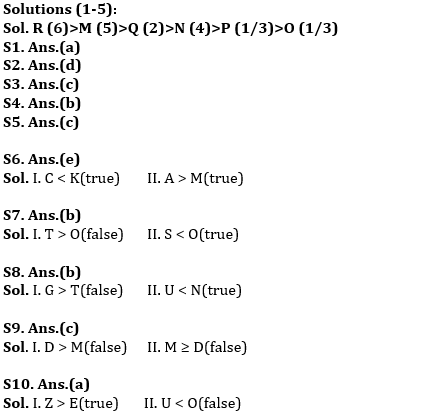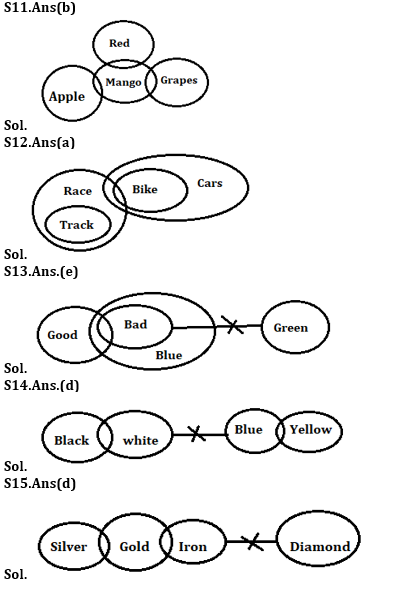Latest Banking jobs   »   Reasoning Ability Quiz For ESIC- UDC,...

# Reasoning Ability Quiz For ESIC- UDC, Steno, MTS Prelims 2022- 31st January

Directions (1-5): Study the following information and answer the given questions.
There are six boxes having different weight. Each box contains different number of candies- 2, 3, 4, 5, 6 and 1 but not necessarily in the same order.
R contains more candies than M and R’s weight more than O, which contains odd number of candies. Box N contains 4 candies. P weighs more than only O. M weighs more than Q and N, but less than R. Box Q contains 2 candies and Q’s weight is more than N. The difference between the number of candies of box M and N is 1 and Box M contains more than 3 candies.

Q1. Which of the following box contains highest number of candies?
(a) R
(b) Q
(c) O
(d) M
(e) None of these

Q2. Which of the following box is third heaviest box?
(a) P
(b) O
(c) R
(d) Q
(e) None of these

Q3. What is the difference between number of candies between box R and Q?
(a) 3
(b) 1
(c) 4
(d) 2
(e) None of these

Q4. Which of the following box is heavier than N but lighter than M?
(a) O
(b) Q
(c) R
(d) P
(e) None of these

Q5. How many boxes are lighter than Q?
(a) One
(b) Two
(c) Three
(d) More than Three
(e) None

Directions (6-10): In these questions, relationship between different elements is shown in the statements. These statements are followed by two conclusions.
(a) If only conclusion I follows.
(b) If only conclusion II follows.
(c) If either conclusion I or II follows.
(d) If neither conclusion I nor II follows.
(e) If both conclusions I and II follow.

Q6. Statements: A>K>D>L, M≤C≤D, L>Q
Conclusions: I. C < K
II. A > M

Q7. Statements: T ≥ M ≥ E, E<O, A>E>S
Conclusions: I. T > O
II. S < O

Q8. Statements: G≥C>U, P=W≥U, W<N<T
Conclusions: I. G > T
II. U < N

Q9. Statements: R>Q>J>D, M≤C≤J
Conclusions: I. D > M
II. M ≥ D

Q10. Statements: Z≥V>U, R=E<U, E>M<O
Conclusions: I. Z > E
II. U < O

Directions (11-15): In each of the questions below are given some statements followed by some Conclusions. You have to take the given statements to be true even, if they seem to be at variance from commonly known facts. Read all the conclusions and then decide which of the given conclusions logically follows from the given statements disregarding commonly known facts.
(a) If only conclusion I follows.
(b) If only conclusion II follows.
(c) If either conclusion I or II follows.
(d) If neither conclusion I nor II follows.
(e) If both conclusions I and II follow.

Q11. Statements:
Only few apple are mango
Some mango are grapes
Only a few mango are Red
Conclusion:
I: Some Red are grapes
II: All grapes can be mango

Q12. Statements:
Only Race are track
Only a few race are bike
All bike are cars
Conclusion:
I: Some cars are race
II: Some track are car is possibility

Q13. Statements:
Conclusions:
I. All good can never be green
II. Some blue are not green

Q14. Statements:
Some black are white
No white is blue
Some blue are yellow
Conclusions:
I. Some yellow are white
II. All black can never be yellow

Q15. Statements:
Only a few silver are gold
Some gold are iron
No iron is diamond
Conclusions:
I: All gold can be diamond
II: Some iron are silver

Solutions#### Congratulations!Union Budget 2023-24: Free PDF